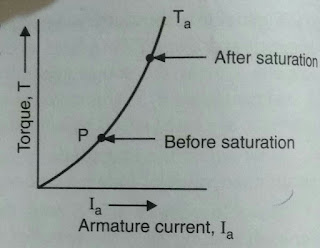# Characteristics of DC Series Motor

0
89

## Characteristics of DC Series Motor

### 1.SPEED – CURRENT CHARACTERISTICS.

#### Since the speed of dc motor is given by;N= k(E/Φ) , where k= proportionality constant.N = k{(V – IR)/Φ}The resistance of armature is very small so voltage drop (IR) can be neglected and supply voltage is constant. So speed is inversely proportional to flux.Now, in dc series motor the flux is produced by the armature current flowing in field winding. So flux produced is directly proportional to the armature current. Hence speed of series motor becomes inversely proportional to the armature current. The curve between them is rectangular hyperbola.### 2. TORQUE- CURRENT CHARACTERISTICS

#### Torque in case of dc motor is directly proportional to the product of flux and armature current.But in dc series motor, flux is directly proportional to the armature current upto saturation. Hence, torque become directly proportional to the square of armature current upto saturation.Hence, the curve is parabola upto saturation. After saturation flux becomes independent of armature current and hence torque become proportional to armature current only.#### Fig. Torque – Current characteristicsThus, dc series motor has very high starting torque.